Function Repository Resource:

# BrocardInellipse

Generate the Brocard inellipse of a 2D triangle

Contributed by: Jan Mangaldan
 ResourceFunction["BrocardInellipse"][{p1,p2,p3}] returns an Ellipsoid representing the Brocard inellipse of the triangle defined by vertices p1,p2, and p3. ResourceFunction["BrocardInellipse"][{p1,p2,p3},property] gives the value of the specified property.

## Details

The Brocard inellipse of a triangle is the unique ellipse inscribed in a triangle whose foci are the Brocard points.
The following properties are supported:
 "Ellipsoid" Ellipsoid representing the inellipse "Parametric" parametric equation for the inellipse as a pure function "Implicit" implicit Cartesian equation for the inellipse as a pure function
ResourceFunction["BrocardInellipse"][poly] where poly is a Triangle or Polygon is equivalent to ResourceFunction["BrocardInellipse"][PolygonCoordinates[poly]].

## Examples

### Basic Examples (1)

Show a triangle together with its Brocard inellipse:

 In:=Out=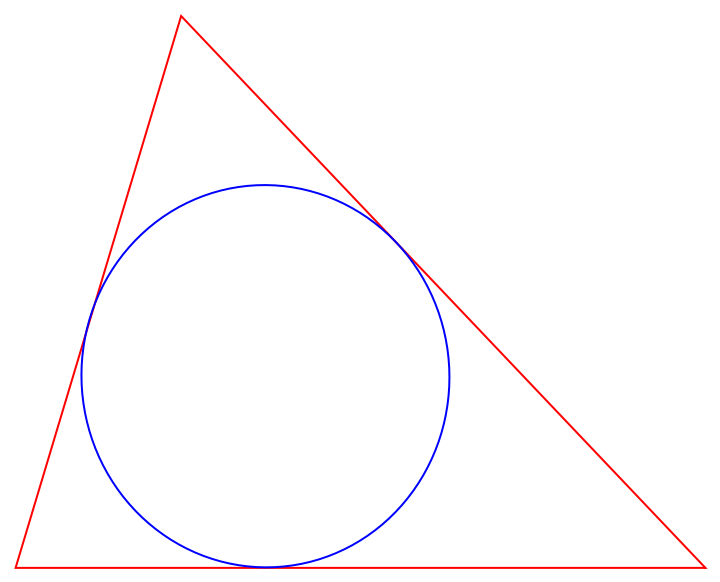### Scope (5)

A triangle:

 In:=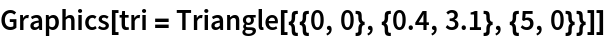Out=Generate the parametric equation of the triangle's Brocard inellipse:

 In:=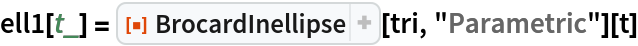Out=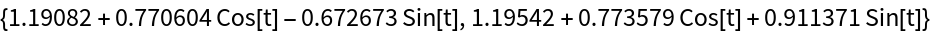Plot the parametric equation along with the triangle:

 In:=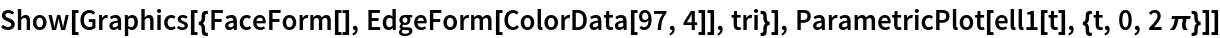Out=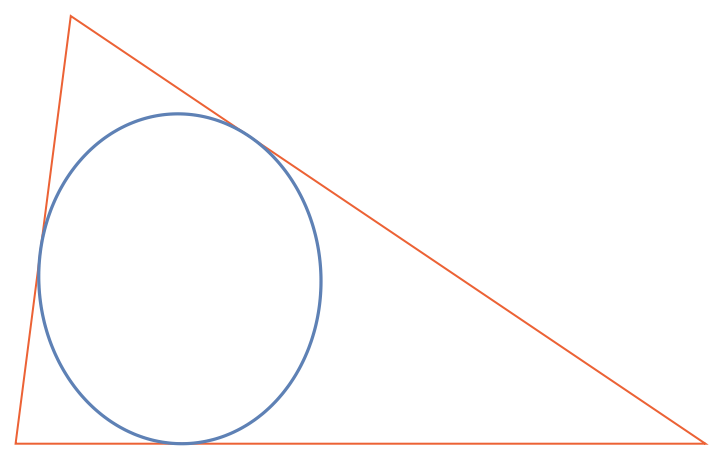Generate the implicit equation of the triangle's Brocard inellipse:

 In:=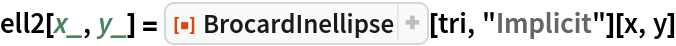Out=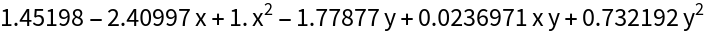Plot the implicit equation along with the triangle:

 In:=Out=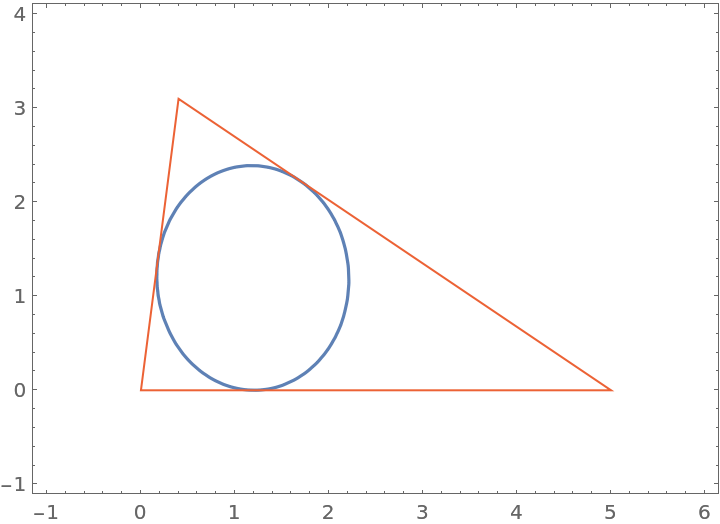### Properties and Relations (2)

Use the resource function EllipseProperties to generate properties of the inellipse:

 In:=Out=The foci of the inellipse are the Brocard points of the triangle:

 In:=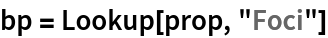Out=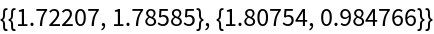Show that the angles formed by the Brocard points and the triangle's vertices are all equal to the Brocard angle:

 In:=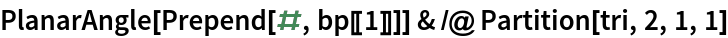Out=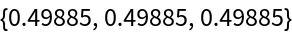In:=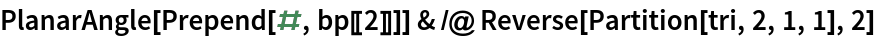Out=In:=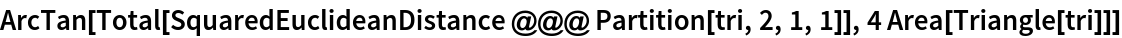Out=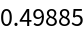The area of the Brocard inellipse can be expressed in terms of the area of the original triangle and the lengths of its sides:

 In:=Out=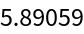In:=Out=## Version History

• 1.0.1 – 24 August 2021